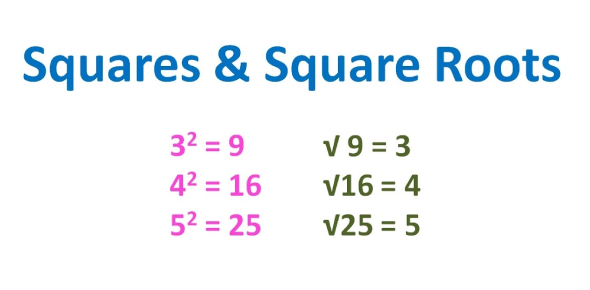# Squares And Square Roots Quiz Questions And Answers

10 Questions | Total Attempts: 13598SettingsTake this amazing squares and square roots quiz. A square is when you multiply a number by itself while on the other hand, a square root is an exact opposite of a square. This means that to find a square root of a given number you have to find the number which when multiplied by itself gives our desired result. Read and answer all the questions given below to obtain your accurate result. Take up the quiz below and revise and sharpen your ability on the subject. Let all your friends know about this quiz!

• 1.
The area of a square is 144 square meters.  Find the perimeter of the square.
• A.

36 m

• B.

24 m

• C.

72 m

• D.

48 m

• 2.
Emma's bedroom is shaped like a square.  What are the dimensions of the room if the area of the floor is 196 square feet?
• A.

49 ft x 49 ft

• B.

7 ft x 7 ft

• C.

14 ft x 14 ft

• D.

4 ft x 49 ft

• 3.
A square garden has an area of 225 square feet.  How much fencing will a gardener need to buy to place fencing around the garden?
• A.

56.25 ft

• B.

112.5 ft

• C.

15 ft

• D.

60 ft

• 4.
What is the square of 7?
• A.

49

• B.

14

• C.

28

• D.

3.5

• 5.
• A.

A

• B.

B

• C.

C

• D.

D

• 6.
• A.

A

• B.

B

• C.

C

• D.

D

• 7.
The area of a square is 100 square meters.  What is the length of one side of the square?
• A.

25

• B.

10

• C.

50

• 8.
• A.

A

• B.

B

• C.

C

• D.

D

Related TopicsBack to top